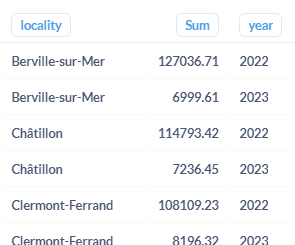# Using field whose name is computed from a field filter

I'm struggling with the following issue related to a native query on MongoDB : I have a collection containing a locality, a year (in number format) and an amount. I make a native query using a filter 'yearFilter' (of type number) on the year, and the filter selects the documents matching the year provided and the previous year. Then I do a sum of amounts grouped by locality and year, which gives me something like this (in this example I provided the value 2023 in the filter, so I have the sums per locality and per year for 2023 and 2022):Then I apply some transformations so that the years become new fields (it's like if I had used the pivot option on the year, in the visualization settings). At the end I have this:So far so good ! Now the tricky part... I would like to add a new column containing the difference between the sum of 2023 and the sum of 2022. If I simply just do this, it works fine:

``````  {
"diff": { \$subtract: ["\$2023", "\$2022"]}
}
},
``````

The problem is that I cannot hardcode the field names like I did, since they depend on the value that I provided in the filter at the beginning. Basically, the field "2023" corresponds to {{yearFilter}}, and the field "2022" corresponds to {\$subtract: [ {{yearFilter}}, 1] }.
So I tried to replace in the code above:

``````  {
"diff": { \$subtract: ["\${{yearFilter}}", "\${\$subtract: [ {{yearFilter}}, 1] }"]}
}
},
``````

But it doesn't work. I see the new column, but it is empty. In order to understand where the problem comes from, I did the following:

``````  {
"YEAR1": "\${{yearFilter}}"
}
},
{
"YEAR2-test1": {\$toString: {\$subtract: [ {{yearFilter}}, 1] } }
}
},
{